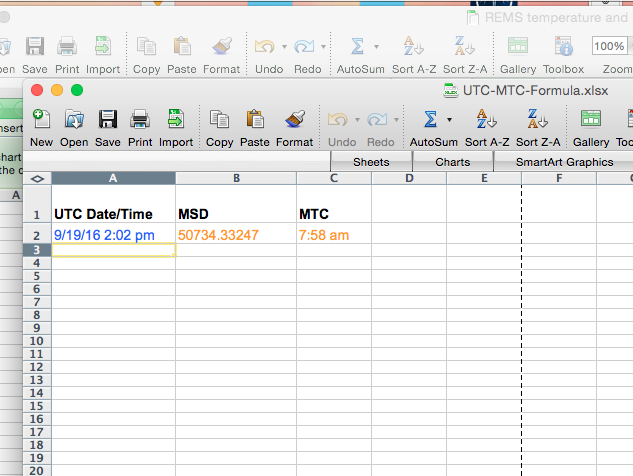# UTC to MTC Conversion in Excel

When it comes to manipulating data on Mars, one of the most difficult problems that we often come into is the inconsistencies in timekeeping standards in NASA’s data. Perhaps the most difficult of these is converting between UTC and MTC timers in order to calculate the significance of certain types of data such as temperature and pressure in correlation with the time of day they are found at. Below, we will show you how to calculate MTC in Excel in order to overcome this.

### Calculating MTC

Step 1) Create a Date/Time in an excel cell and rename the cell to “UTC”.

Step 2) Insert the following formula into another cell:

`=TIME(FLOOR(MOD(((((UTC*86400 - (DATE(1970,1,1)*86400))/86400+2440587.5+(67.184/86400)-2451545-4.5)/1.027491252)+44796-0.00096)*24, 24),1),FLOOR(MOD(((((UTC*86400 - (DATE(1970,1,1)*86400))/86400+2440587.5+(67.184/86400)-2451545-4.5)/1.027491252)+44796-0.00096)*1440, 60),1),FLOOR(MOD(((((UTC*86400 - (DATE(1970,1,1)*86400))/86400+2440587.5+(67.184/86400)-2451545-4.5)/1.027491252)+44796-0.00096)*86400, 60),1)) `

This will then return the time in Mars Coordinated Time.

### Calculating MSD

Step 1) Create a Date/Time in an excel cell and rename the cell to “UTC”.

Step 2) Insert the following formula into another cell:

`=((UTC*86400 - (DATE(1970,1,1)*86400))/86400+2440587.5+(67.184/86400)-2451545-4.5)/1.027491252+44796-0.00096`

This will then return the time in Mars Solar Date.

You can download an excel file with examples of each here: UTC/MTC Formula

Remember, you will still have to correct for the Local Mean Solar time of whatever destination your data is collected from. This information is generally available from the Wikipedia pages of each individual mission.

### Dean Little

Dean is an astrophysicist, hardware and software engineer and Mars enthusiast who develops engineering solutions for the red planet.
Scroll to top